AP State Syllabus AP Board 7th Class Maths Solutions Chapter 4 Lines and Angles Ex 4 Textbook Questions and Answers.

## AP State Syllabus 7th Class Maths Solutions 4th Lesson Lines and Angles Exercise 4Question 1.
Which of the following are adjacent angles?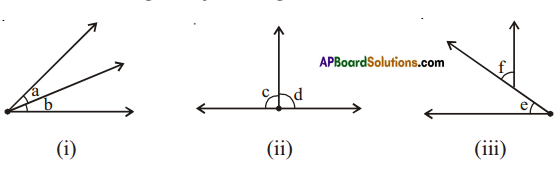Solution:
(i)
i) a and b are adjacent angles.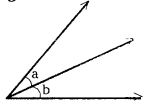ii) c and d are adjacent angles.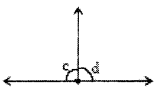iii) e, f are not adjacent angles.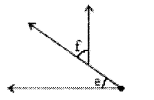Question 2.
Name all pairs of adjacent angles in the figure. How many pairs of adjacent angles are formed? Why these angles are called adjacent angles?
Solution:
(∠AOL). ∠AOC). (∠AOC. ∠BOC)
(∠BOC. ∠BOD), (∠BOD. ∠AOD) are the four pairs of adjacent angles.
These are adjacent since they have a common vertex, common arm and on either sides of the common arm.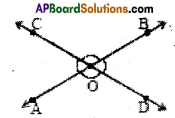Question 3.
Can two adjacent angles be supplementary? Draw figure.
Solution:
Yes. Two adjacent angles can be supplementary.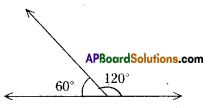Question 4.
Can two adjacent angles be complementary? Draw figure.
Solution: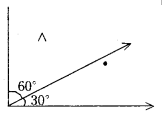Yes. Two adjacent angles can be complementary.Question 5.
Give four examples of adj acent angles in daily life.
Example: Angles between the spokes at the centre ofa cycle wheel.
(i) ____________________________
(ii) ____________________________
(iii) ____________________________
(iv) ____________________________
Solution:
i) Angles between (Door and Wall). (Door and frame)
ii) Angles between the 3 hands of a clock at a point of time.
iii) Adjacent angles in the Rangoli.
iv) Angles between spokes of the window design.